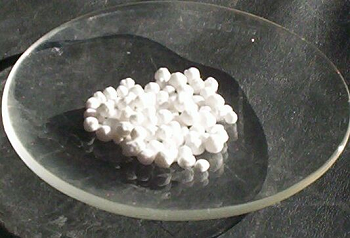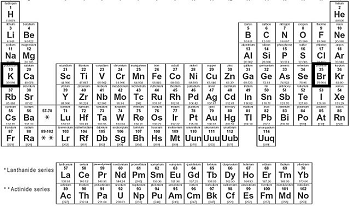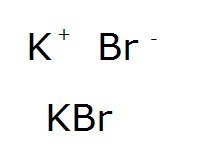# Potassium Bromide: Formula & Side Effects

Instructor: Sarah Erhart

Sarah has taught college physical, organic, and general chemistry and high school biology. She has a master's degree in chemistry.

In this lesson, you will learn about potassium bromide. We will review the basics, describe how the formula and weight are determined, and depict some of its common uses.

## What Is Potassium Bromide?

Potassium bromide, under normal pressure and temperature, is a white crystalline salt (Figure 1).It is soluble in water, which means it will easily dissociate into free ions and will appear to 'disappear' when put in water. At a particulate level, the crystal is arranged in an alternating pattern of potassium and chloride ions (Figure 2).## Formula and Weight

Potassium bromide is an ionic compound. It is labeled an ionic compound due to the type of bonding that occurs between the two elements - an electrostatic attraction between the two ions. One can usually predict ionic bonding based on the elements' position on the periodic table. A metal with a non-metal will almost always have ionic bonding. Figure 3 shows the periodic table with potassium and bromide highlighted. Potassium is a metal and bromide is a non-metal.The periodic table is also useful for determining the formula of potassium bromide. The column in which the element is located tells us something about the charge that the element commonly has. Since potassium is in the first column, we know that it is usually charged +1. Bromine is in the column that is one to the left of the noble gasses, or two to the left of the end, which means it is commonly charged -1. Most compounds, potassium bromide included, are neutral; therefore, to have a neutral compound the formula for potassium bromide must be KBr (Figure 4).

Finally, the periodic table can also be used to determine the formula weight of the salt. Potassium has a mass of 39.1 g/mol and bromide 79.9 g/mol; adding these together gives a formula mass of 119 g/mol for potassium bromide.To unlock this lesson you must be a Study.com Member.

### Register to view this lesson

Are you a student or a teacher?